# An Approach to Mathematic Functions Basics

## Section XLII - The Tangent Addition Formula in Action

### Summary for the Section :

• In this Section , we shall attempt to express :

• the { tangent of [ N * Theta ] } in terms of the { tangent of [ Theta ] } .

### Setting-up the value [ T ] as the { tangent of [ Theta ] } :

• Let us first set up [ T ] as the value of the { tangent of [ Theta ] } , i.e. :

•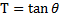And we bring-in again the Tangent Addition Formula :

•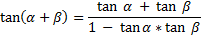for our applications below .

### The Tangent Addition Formula in action :

• First-of-all , for the { tangent of [ 2*Theta ] } :

• we can simply substitute into the Tangent Addition Formula :

• { [ Alpha ] = [ Theta ] } and { [ Beta ] = [ Theta ] }

to arrive at :

•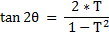• And :

• For the { Tangent of [ 3*Theta ] } , we can use :

• { [ Alpha ] = [ 2*Theta ] } and { [ Beta ] = [ Theta ] }

to arrive at :

•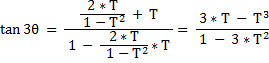• For the { Tangent of [ 4*Theta ] } , we can use :

• { [ Alpha ] = [ 3*Theta ] } and { [ Beta ] = [ Theta ] }

to arrive at :

•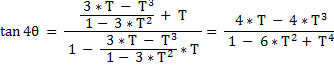• For the { Tangent of [ 5*Theta ] } , we can use :

• { [ Alpha ] = [ 4*Theta ] } and { [ Beta ] = [ Theta ] }

to arrive at :

•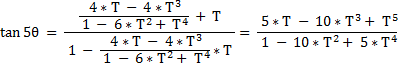• For the { Tangent of [ 6*Theta ] } , we can use :

• { [ Alpha ] = [ 5*Theta ] } and { [ Beta ] = [ Theta ] }

to arrive at :

•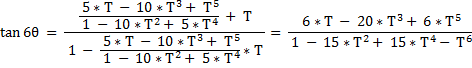• For the { Tangent of [ 7*Theta ] } , we can use :

• { [ Alpha ] = [ 6*Theta ] } and { [ Beta ] = [ Theta ] }

to arrive at :

•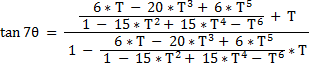yielding :

•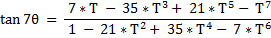• For the { Tangent of [ 8*Theta ] } , we can use :

• { [ Alpha ] = [ 7*Theta ] } and { [ Beta ] = [ Theta ] }

to arrive at :

•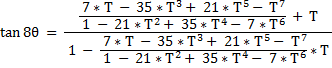yielding :

•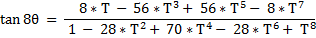### Summarizing :

• Let us now quickly summarize the results of our calculation process above .

And we have :

•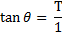••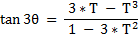•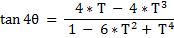•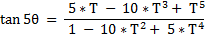•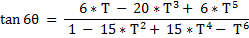••• QUESTION :

• Do we see a pattern here ?

And

• the ANSWER is yes !

More details on this in the next Section coming up .